### IMO Shortlist 1997 problem 1

Kvaliteta:
Avg: 0,0
Težina:
Avg: 0,0
In the plane the points with integer coordinates are the vertices of unit squares. The squares are coloured alternately black and white (as on a chessboard). For any pair of positive integers$m$ and$n$, consider a right-angled triangle whose vertices have integer coordinates and whose legs, of lengths$m$ and$n$, lie along edges of the squares. Let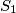$S_1$ be the total area of the black part of the triangle and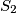$S_2$ be the total area of the white part. Let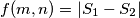$f(m,n) = | S_1 - S_2 |$.

a) Calculate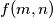$f(m,n)$ for all positive integers$m$ and$n$ which are either both even or both odd.

b) Prove that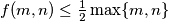$f(m,n) \leq \frac 12 \max \{m,n \}$ for all$m$ and$n$.

c) Show that there is no constant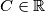$C\in\mathbb{R}$ such that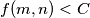$f(m,n) < C$ for all$m$ and$n$.
Izvor: Međunarodna matematička olimpijada, shortlist 1997